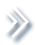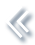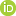####ArchivesReview Articles

## Shape-constrained semiparametric additive stochastic volatility models

Jiangyong Yin ,

Department of Statistics, The Ohio State University, Columbus, OH, USAPeter F. Craigmile ,

Department of Statistics, The Ohio State University, Columbus, OH, USAXinyi Xu ,

Department of Statistics, The Ohio State University, Columbus, OH, USAxu.214@osu.edu

Steven MacEachern

Department of Statistics, The Ohio State University, Columbus, OH, USAPages 71-82 | Received 04 Jun. 2018, Accepted 20 Jan. 2019, Published online: 04 Feb. 2019,
• Abstract
• References
• Citations

ABSTRACT

Nonparametric stochastic volatility models, although providing great flexibility for modelling the volatility equation, often fail to account for useful shape information. For example, a model may not use the knowledge that the autoregressive component of the volatility equation is monotonically increasing as the lagged volatility increases. We propose a class of additive stochastic volatility models that allow for different shape constraints and can incorporate the leverage effect – asymmetric impact of positive and negative return shocks on volatilities. We develop a Bayesian fitting algorithm and demonstrate model performance on simulated and empirical datasets. Unlike general nonparametric models, our model sacrifices little when the true volatility equation is linear. In nonlinear situations we improve the model fit and the ability to estimate volatilities over general, unconstrained, nonparametric models.

## References

1. Andrieu, C., Doucet, A., & Holenstein, R. (2010). Particle Markov chain Monte Carlo methods. Journal of the Royal Statistical Society: Series B (Statistical Methodology)72, 269–342. doi: 10.1111/j.1467-9868.2009.00736.x [Crossref][Web of Science ®], [Google Scholar]
2. Bauwens, L., & Veredas, D. (2004). The stochastic conditional duration model: A latent variable model for the analysis of financial durations. Journal of Econometrics119, 381–412. doi: 10.1016/S0304-4076(03)00201-X [Crossref][Web of Science ®], [Google Scholar]
3. Brezger, A., & Steiner, W. J. (2008). Monotonic regression based on Bayesian P–splines. Journal of Business & Economic Statistics26, 90–104. doi: 10.1198/073500107000000223 [Taylor & Francis Online][Web of Science ®], [Google Scholar]
4. Cai, B., & Dunson, D. (2007). Bayesian multivariate isotonic regression splines. Journal of the American Statistical Association102, 1158–1171. doi: 10.1198/016214506000000942 [Taylor & Francis Online][Web of Science ®], [Google Scholar]
5. Chib, S., & Greenberg, E. (1994). Bayes inference in regression models with ARMA[Math Processing Error](p,q) errors. Journal of Econometrics64, 183–206. doi: 10.1016/0304-4076(94)90063-9 [Crossref][Web of Science ®], [Google Scholar]
6. Chib, S., & Greenberg, E. (1995). Understanding the Metropolis-Hastings algorithm. The American Statistician49, 327–335. [Taylor & Francis Online][Web of Science ®], [Google Scholar]
7. Comte, F. (2004). Kernel deconvolution of stochastic volatility models. Journal of Time Series Analysis25, 563–582. doi: 10.1111/j.1467-9892.2004.01825.x [Crossref][Web of Science ®], [Google Scholar]
8. Eddelbuettel, D., & Sanderson, C. (2014). RcppArmadillo: Accelerating R with high-performance C++ linear algebra. Computational Statistics & Data Analysis71, 1054–1063. doi: 10.1016/j.csda.2013.02.005 [Crossref][Web of Science ®], [Google Scholar]
9. Engle, R. (1982). Autoregressive conditional heteroscedasticity with estimates of the variance of United Kingdom inflation. Econometrica50, 987–1007. doi: 10.2307/1912773 [Crossref][Web of Science ®], [Google Scholar]
10. Engle, R., & Gonzalez-Rivera, G. (1991). Semiparametric ARCH models. Journal of Business & Economic Statistics9, 345–359. [Taylor & Francis Online][Web of Science ®], [Google Scholar]
11. Engle, R., & Ng, V. (1993). Measuring and testing the impact of news on volatility. The Journal of Finance48, 1749–1778. doi: 10.1111/j.1540-6261.1993.tb05127.x [Crossref][Web of Science ®], [Google Scholar]
12. Godsill, S. J., Doucet, A., & West, M. (2004). Monte Carlo smoothing for nonlinear time series. Journal of the American Statistical Association99, 156–168. doi: 10.1198/016214504000000151 [Taylor & Francis Online][Web of Science ®], [Google Scholar]
13. Gordon, N. J., Salmond, D. J., & Smith, A. F. (1993). Novel approach to nonlinear/non-Gaussian Bayesian state estimation. IEEE Proceedings F (Radar and Signal Processing)140, 107–113. doi: 10.1049/ip-f-2.1993.0015 [Crossref][Web of Science ®], [Google Scholar]
14. Grenander, U., & Miller, M. I. (1994). Representations of knowledge in complex systems. Journal of the Royal Statistical Society. Series B (Methodological)56, 549–603. doi: 10.1111/j.2517-6161.1994.tb02000.x [Crossref][Web of Science ®], [Google Scholar]
15. Harvey, A. C., & Shephard, N. (1996). Estimation of an asymmetric stochastic volatility model for asset returns. Journal of Business & Economic Statistics14, 429–434. [Taylor & Francis Online][Web of Science ®], [Google Scholar]
16. Hull, J., & White, A. (1987). The pricing of options on assets with stochastic volatilities. The Journal of Finance42, 281–300. doi: 10.1111/j.1540-6261.1987.tb02568.x [Crossref][Web of Science ®], [Google Scholar]
17. Kim, S., Shephard, N., & Chib, S. (1998). Stochastic volatility: Likelihood inference and comparison with ARCH models. The Review of Economic Studies65, 361–393. doi: 10.1111/1467-937X.00050 [Crossref][Web of Science ®], [Google Scholar]
18. Liu, J. S., & Chen, R. (1998). Sequential Monte Carlo methods for dynamic systems. Journal of the American Statistical Association93, 1032–1044. doi: 10.1080/01621459.1998.10473765 [Taylor & Francis Online][Web of Science ®], [Google Scholar]
19. Meyer, M. C., Hackstadt, A. J., & Hoeting, J. A. (2011). Bayesian estimation and inference for generalised partial linear models using shape-restricted splines. Journal of Nonparametric Statistics23, 867–884. doi: 10.1080/10485252.2011.597852 [Taylor & Francis Online][Web of Science ®], [Google Scholar]
20. Nakajima, J., & Omori, Y. (2009). Leverage, heavy-tails and correlated jumps in stochastic volatility models. Computational Statistics & Data Analysis53, 2335–2353. doi: 10.1016/j.csda.2008.03.015 [Crossref][Web of Science ®], [Google Scholar]
21. Neelon, B., & Dunson, D. (2004). Bayesian isotonic regression and trend analysis. Biometrics60, 398–406. doi: 10.1111/j.0006-341X.2004.00184.x [Crossref][Web of Science ®], [Google Scholar]
22. Nelson, D. (1991). Conditional heteroskedasticity in asset returns: A new approach. Econometrica59, 347–370. doi: 10.2307/2938260 [Crossref][Web of Science ®], [Google Scholar]
23. Omori, Y., Chib, S., Shephard, N., & Nakajima, J. (2007). Stochastic volatility with leverage: Fast and efficient likelihood inference. Journal of Econometrics140, 425–449. doi: 10.1016/j.jeconom.2006.07.008 [Crossref][Web of Science ®], [Google Scholar]
24. R Core Team (2013). R: A Language and Environment for Statistical Computing. R Foundation for Statistical Computing, Vienna, Austria. URL http://www.R-project.org/. [Google Scholar]
25. Ramsay, J. (1988). Monotone regression splines in action. Statistical Science3, 425–441. doi: 10.1214/ss/1177012761 [Crossref], [Google Scholar]
26. Roberts, G. O., & Tweedie, R. L. (1996). Exponential convergence of Langevin distributions and their discrete approximations. Bernoulli2, 341–363. doi: 10.2307/3318418 [Crossref], [Google Scholar]
27. Rydberg, T. (2000). Realistic statistical modelling of financial data. International Statistical Review68, 233–258. doi: 10.1111/j.1751-5823.2000.tb00329.x [Crossref][Web of Science ®], [Google Scholar]
28. Taylor, S. (1994). Modeling stochastic volatility: A review and comparative study. Mathematical Finance4, 183–204. doi: 10.1111/j.1467-9965.1994.tb00057.x [Crossref], [Google Scholar]
29. Yu, J. (2005). On leverage in a stochastic volatility model. Journal of Econometrics127, 165–178. doi: 10.1016/j.jeconom.2004.08.002 [Crossref][Web of Science ®], [Google Scholar]
30. Yu, J. (2012). A semiparametric stochastic volatility model. Journal of Econometrics167, 473–482. doi: 10.1016/j.jeconom.2011.09.029 [Crossref][Web of Science ®], [Google Scholar]